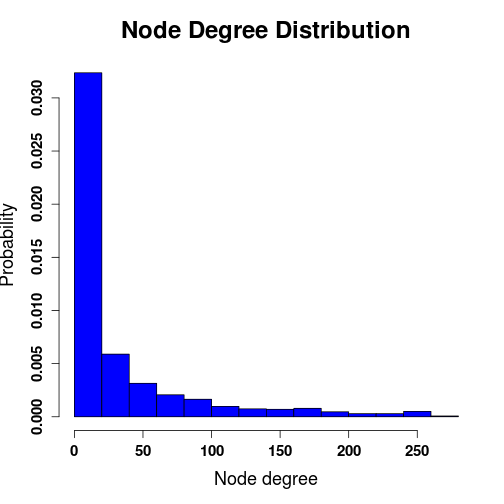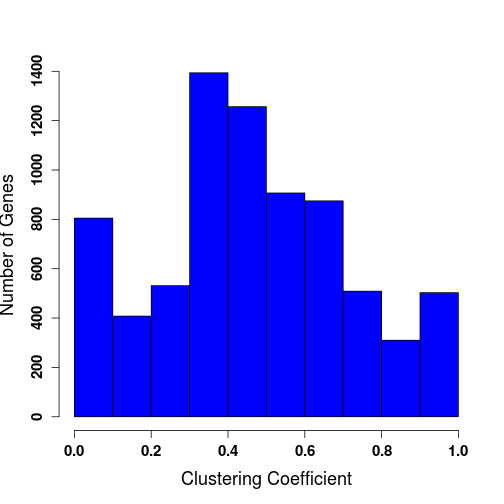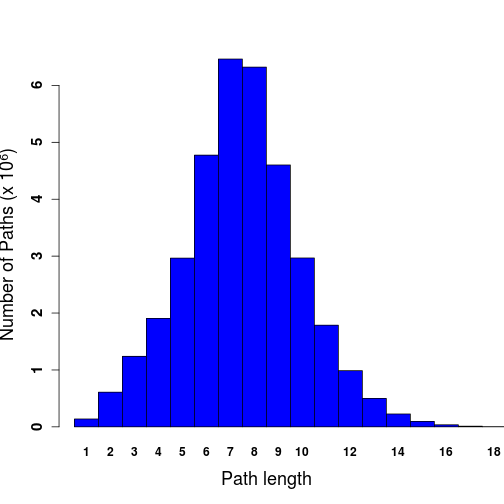Topological Analysis of ChlamyNET

The R package igraph provides a set of functions for the analysis of networks.

``````library("igraph")
``````

The function read.graph is used to load ChlamyNET from a file in gml format.

``````setwd("~/Documents/ChlamyNET_data")
chlamynet
``````
``````## IGRAPH U--- 8443 138575 --
## + attr: root_index (v/n), id (v/n), graphics (v/c), label (v/c),
##   root_index (e/n), graphics (e/c), label (e/c)
``````

The degree of a node or gene represents the number of neighbouring nodes or co-expressed genes. The function degree.distribution returns the degree distribution of a network. The function power.law.fit fits a data set to a power-law distribution. It is a complementary test to the one based on linear regression to determine if a network is scale-free.

``````network.degree.distribution <- degree.distribution(chlamynet)
fit.scale.free <- power.law.fit(network.degree.distribution)
fit.scale.free[["KS.p"]]
``````
``````##  0.9993257
``````

A high p-value indicates that ChlamyNET is a scale-free network. The following histogram represents the degree distribution of ChlamyNET.

``````network.degrees <- degree(chlamynet)
degree.histogram <- hist(network.degrees,freq=FALSE,col="blue",xlab="Node degree", ylab="Probability",main="Node Degree Distribution",cex.axis=1.25,font.axis=2,cex.main=2,cex.lab=1.5)
``````A data frame is created to store the degree of every node. These data is then saved into a file than can be loaded into Cytoscape:

``````node.degree.data <- data.frame(V(chlamynet)\$label,network.degrees)
colnames(node.degree.data) <- c("name","degree")
write.table(node.degree.data,file="chlamy_degree.txt",quote=FALSE,col.names=TRUE,row.names=FALSE)
``````

The clustering coefficient or transitivity of a given node measures the tendency of its neighbouring nodes to group together among them. When applied to gene co-expression networks the clustering coefficient measures the degree of co-expression among the genes co-expressed with a given gene. The function transitivity with the argument type set to global computes the mean clustering coefficient of a given network:

``````chlamynet.transitivity <- transitivity(chlamynet,type="global") #0.6577137 0.66
chlamynet.transitivity
``````
``````##  0.6577137
``````

The function transitivity with the argument type set to local computes the clustering coefficient of every node in the given network. A data frame is created to store the clustering coefficient of every node. These data is then saved into a file than can be loaded into Cytoscape:

``````clustering.coefficient <- transitivity(chlamynet,type="local",isolates="NaN")
clustering.coefficient.data <- data.frame(V(chlamynet)\$label,clustering.coefficient)
colnames(clustering.coefficient.data) <- c("name","clustering_coefficient")
write.table(clustering.coefficient.data,file="chlamy_clustering_coefficient.txt",quote=FALSE,col.names=TRUE,row.names=FALSE)
``````

The distribution of the clustering coefficient can be represented using the following histogram:

``````hist(clustering.coefficient,col="blue",xlab="Clustering Coefficient",ylab="Number of Genes",cex.axis=1.25,font.axis=2,cex.main=2,cex.lab=1.5,main="")
``````A small world network is a free-scale network with a clustering coefficient significantly high when compared to random free-scale networks. In order to check whether or not ChlamyNET is a small world network we generate 10000 random free-sacle networks with the same number of nodes and edges as ChlamyNET and compare their clustering coefficient to the one of ChlamyNET.

A binomial distribution with probability prob will be used to generate the random networks. The function barabasi.game is used to generate random free-scale networks.

``````chlamynet
``````
``````## IGRAPH U--- 8443 138575 --
## + attr: root_index (v/n), id (v/n), graphics (v/c), label (v/c),
##   root_index (e/n), graphics (e/c), label (e/c)
``````
``````prob <- (138575/8443)/30

random.transitivity <- vector(length=10000,mode="numeric")

for(i in 1:10000)
{
edges.vector <- rbinom(n=8443,size=30,prob=prob)
random.network <- barabasi.game(n=8443,out.seq=edges.vector,directed=TRUE)
random.transitivity[i] <- transitivity(random.network,type="global",isolates="zero")
}

estimated.p.value <- sum(random.transitivity > chlamynet.transitivity) / 10000
estimated.p.value
``````
``````##  0
``````

As the estimated p value shows ChlamyNET is a small world network. Next, we compute the average minimum path length between nodes in ChlamyNET and represent the corresponding distribution using a histogram.

``````average.path.length(chlamynet, directed=FALSE, unconnected=TRUE)
``````
``````##  7.479656
``````
``````path.length.chlamynet <- path.length.hist(chlamynet, directed=FALSE)
path.length.chlamy.values <- path.length.chlamynet[["res"]]
names(path.length.chlamy.values) <- 1:length(path.length.chlamy.values)

barplot(path.length.chlamy.values/1e6,space=0,col="blue",xlim=c(0,16),xlab="Path length",ylab="Number of Paths (x 10⁶)",cex.axis=1.25,font.axis=2,cex.main=2,cex.lab=1.5)
``````
``````## Warning in title(main = main, sub = sub, xlab = xlab, ylab = ylab, ...):
## conversion failure on 'Number of Paths (x 10⁶)' in 'mbcsToSbcs': dot
## substituted for <e2>
``````
``````## Warning in title(main = main, sub = sub, xlab = xlab, ylab = ylab, ...):
## conversion failure on 'Number of Paths (x 10⁶)' in 'mbcsToSbcs': dot
## substituted for <81>
``````
``````## Warning in title(main = main, sub = sub, xlab = xlab, ylab = ylab, ...):
## conversion failure on 'Number of Paths (x 10⁶)' in 'mbcsToSbcs': dot
## substituted for <b6>
``````In free-scale networks, nodes with high degrees play a key role on the robustness of the network and on the propagation of information across the network. These nodes are called hubs. Next we determine the 1000 nodes with the highest degree in ChlamyNET and save them in a file for subsequent analysis.

``````node.degree <- degree(chlamynet)
names(node.degree) <- V(chlamynet)\$label
sorted.node.degree <- sort(node.degree,decreasing=TRUE)
hubs.1000 <- names(sorted.node.degree[1:1000])
write(hubs.1000,file="hubs_1000.txt")
``````

The definition of hubs solely based on the node degree has been argued to be incomplete and the concept of authoritative hub was introduced. An authoritative hub should not ony have a large degree. Additionally, its neighbouring nodes should in turn establish links between them. We identified the first 1000 authoritative hubs using the HITS algorithm using the hub.score function and save them in a file for subsequent analysis.

``````chlamynet.hub.scores <- hub.score(chlamynet)
chlamynet.hub.scores.values <- chlamynet.hub.scores[["vector"]]
hub.score.data <- data.frame(V(chlamynet)\$label,chlamynet.hub.scores.values)
colnames(hub.score.data) <- c("name","hub_score")
write.table(hub.score.data,file="chlamy_hub_score.txt",quote=FALSE,col.names=TRUE,row.names=FALSE)
chlamynet.hub.scores.values.sorted <- sort(chlamynet.hub.scores.values,decreasing=TRUE)
write(names(chlamynet.hub.scores.values.sorted[1:1000]),file="hubs_authoritative_1000.txt")
``````